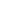Original research

# Estimation of accuracy rate in predicting cardiovascular disease using Gaussian Naive Bayes algorithm with logistic regression

## Abstract

Aim: Comparison of accuracy rate in prediction of cardiovascular disease using Naive Bayes with Logistic Regression. Materials and Methods: The Naive Bayes (N=10) and Logistic Regression Algorithm (N=10) these two algorithms are calculated by using 2 Groups and taken 20 samples for both algorithm and accuracy in this work. The sample size is determined using the G power Calculator and it’s found to be 10. Results: Based on the Results Accuracy obtained in terms of accuracy is identified by Naive Bayes (87.02%) over the Logistic Regression algorithm (92.18%). Statistical significance difference between novel Naive Bayes algorithm and Logistic Regression Algorithm was found to be p=0.001 (2 tailed) (p<0.05). Conclusion: Prediction of cardiovascular disease using Logistic Regression is significantly better than the Naive Bayes.

## Imprint

Talluri Vishnuvardhan, A.Rama. Estimation of Accuracy Rate in Predicting Cardiovascular Disease using Gaussian Naive Bayes Algorithm with Logistic Regression. Cardiometry; Issue 25; December 2022; p.1532-1537; DOI: 10.18137/cardiometry.2022.25.15321537; Available from: https://www.cardiometry.net/issues/no25-december-2022/estimation-accuracy-rate

## Keywords

Novel naive bayes,   Novel logistic regression,   Machine learning,   Healthcare,   Blood Coronary,   VasculitisCurrent issueCardiometry's libraryFounders of Cardiometry